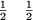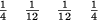## Leibniz Harmonic TriangleIn the Leibniz harmonic triangle, each Fraction is the sum of numbers below it, with the initial and final entry on each row one over the corresponding entry in Pascal's Triangle. The Denominators in the second diagonals are 6, 12, 20, 30, 42, 56, ... (Sloane's A007622).

See also Catalan's Triangle, Clark's Triangle, Euler's Triangle, Number Triangle, Pascal's Triangle, Seidel-Entringer-Arnold Triangle

References

Sloane, N. J. A. Sequence A007622/M4096 in An On-Line Version of the Encyclopedia of Integer Sequences.'' http://www.research.att.com/~njas/sequences/eisonline.html and Sloane, N. J. A. and Plouffe, S. The Encyclopedia of Integer Sequences. San Diego: Academic Press, 1995.